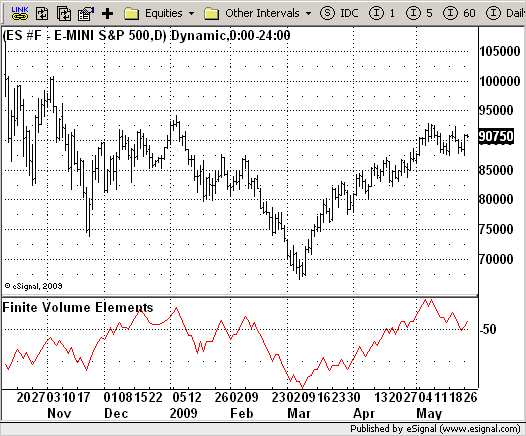# Finite Volume Elements (FVE)

ICE Data Services -

FVE_Katsanos.efs                                                                                      EFSLibrary - Discussion Board

File Name: FVE_Katsanos.efs

Description:
Finite Volume Elements (FVE)

Formula Parameters:
Period : 22
Factor : 0.03

Notes:
The FVE is a pure volume indicator. Unlike most of the other indicators
(except OBV), price change doesn?t come into the equation for the FVE (price
is not multiplied by volume), but is only used to determine whether money is
flowing in or out of the stock. This is contrary to the current trend in the
design of modern money flow indicators. The author decided against a price-volume
indicator for the following reasons:
- A pure volume indicator has more power to contradict.
- The number of buyers or sellers (which is assessed by volume) will be the same,
regardless of the price fluctuation.
- Price-volume indicators tend to spike excessively at breakouts or breakdowns.

FVE_Katsanos.efsEFS Code:

```/*********************************
Provided By:
eSignal (Copyright c eSignal), a division of Interactive Data
Formula Script (EFS) is for educational purposes only and may be
modified and saved under a new file name.  eSignal is not responsible
for the functionality once modified.  eSignal reserves the right
to modify and overwrite this EFS file with each new release.

Description:
Finite Volume Elements (FVE)

Version:            1.0  05/25/2009

Formula Parameters:                     Default:
Period                              22
Factor                              0.03

Notes:
The FVE is a pure volume indicator. Unlike most of the other indicators
(except OBV), price change doesn?t come into the equation for the FVE (price
is not multiplied by volume), but is only used to determine whether money is
flowing in or out of the stock. This is contrary to the current trend in the
design of modern money flow indicators. The author decided against a price-volume
indicator for the following reasons:
- A pure volume indicator has more power to contradict.
- The number of buyers or sellers (which is assessed by volume) will be the same,
regardless of the price fluctuation.
- Price-volume indicators tend to spike excessively at breakouts or breakdowns.

**********************************/
var fpArray = new Array();
var bInit = false;

function preMain() {
setStudyTitle("Finite Volume Elements");
setCursorLabelName("FVI",0);
setDefaultBarFgColor(Color.red,0);
var x = 0;
fpArray[x] = new FunctionParameter("Period", FunctionParameter.NUMBER);
with(fpArray[x++]) {
setLowerLimit(1);
setDefault(22);
}
fpArray[x] = new FunctionParameter("Factor", FunctionParameter.NUMBER);
with(fpArray[x++]) {
setLowerLimit(0.01);
setDefault(0.3);
}
}

var xFVE = null;

function main(Period, Factor) {
var nBarState = getBarState();
var nFVE = 0;
if (nBarState == BARSTATE_ALLBARS) {
if (Period == null) Period = 22;
if (Factor == null) Factor = 0.3;
}
if (bInit == false) {
xFVE = efsInternal("Calc_FVE", Period, Factor);
bInit = true;
}
nFVE = xFVE.getValue(0);
if (nFVE == null) return;
return nFVE;
}

var bSecondInit = false;
var xhl2 = null;
var xhlc3 = null;
var xClose = null;
var xVolume = null;
var xSMAV = null;

function Calc_FVE(Period, Factor) {
var nRes = 0;
var nMF = 0;
var nVlm = 0
var nClose = 0;
var nVolumeMA = 0;
var nRef = ref(-1);
if (bSecondInit == false) {
xhl2 = hl2();
xhlc3 = hlc3();
xClose = close();
xVolume = volume();
xSMAV = sma(Period, xVolume);
bSecondInit = true;
}
nVolumeMA = xSMAV.getValue(0)
nClose = xClose.getValue(0);
if (nVolumeMA == null) return;
nMF = nClose - xhl2.getValue(0) + xhlc3.getValue(0) - xhlc3.getValue(-1);
if(nMF > Factor * nClose / 100)
nVlm = xVolume.getValue(0);
else if(nMF < -Factor * nClose / 100)
nVlm = - xVolume.getValue(0);
else
nVlm = 0;
nRes = nRef + ((nVlm / nVolumeMA) / Period) * 100;
return nRes;
}
```